带通滤波器的参数设置_matlab带通滤波器参数设置 - CSDN

• 下两个滤波器都是切比雪夫I型数字滤波器，不是巴特沃尔滤波器，请使用者注意！1.低通滤波器使用说明：将下列代码幅值然后以m文件保存，文件名要与函数名相同，这里函数名：lowp。function y=lowp(x,f1,f3,rp,rs,Fs) ...
本文为转载内容，原文地址为点击打开链接。下两个滤波器都是切比雪夫I型数字滤波器，不是巴特沃尔滤波器，请使用者注意！1.低通滤波器使用说明：将下列代码幅值然后以m文件保存，文件名要与函数名相同，这里函数名：lowp。function y=lowp(x,f1,f3,rp,rs,Fs)
%低通滤波
%使用注意事项：通带或阻带的截止频率的选取范围是不能超过采样率的一半
%即，f1,f3的值都要小于 Fs/2
%x:需要带通滤波的序列
% f 1：通带截止频率
% f 3：阻带截止频率
%rp：边带区衰减DB数设置
%rs：截止区衰减DB数设置
%FS：序列x的采样频率
% rp=0.1;rs=30;%通带边衰减DB值和阻带边衰减DB值
% Fs=2000;%采样率
%
wp=2*pi*f1/Fs;
ws=2*pi*f3/Fs;
% 设计切比雪夫滤波器；
[n,wn]=cheb1ord(wp/pi,ws/pi,rp,rs);
[bz1,az1]=cheby1(n,rp,wp/pi);
%查看设计滤波器的曲线
[h,w]=freqz(bz1,az1,256,Fs);
h=20*log10(abs(h));
figure;plot(w,h);title('所设计滤波器的通带曲线');grid on;
%
y=filter(bz1,az1,x);%对序列x滤波后得到的序列y
end--------------------------------------低通滤波器使用例子的代码fs=2000;
t=(1:fs)/fs;
ff1=100;
ff2=400;
x=sin(2*pi*ff1*t)+sin(2*pi*ff2*t);
figure;
subplot(211);plot(t,x);
subplot(212);hua_fft(x,fs,1);
%低通测试
% y=filter(bz1,az1,x);
y=lowp(x,300,350,0.1,20,fs);
figure;
subplot(211);plot(t,y);
subplot(212);hua_fft(y,fs,1);%hua_fft()函数是画频谱图的函数，代码在下面给出，要保存为m文件调用%这段例子还调用了我自己写的专门画频谱图的函数，也给出，不然得不出我的结果;%画信号的幅频谱和功率谱
%频谱使用matlab例子表示
function hua_fft(y,fs,style,varargin)
%当style=1,画幅值谱；当style=2,画功率谱;当style=其他的，那么花幅值谱和功率谱
%当style=1时，还可以多输入2个可选参数
%可选输入参数是用来控制需要查看的频率段的
%第一个是需要查看的频率段起点
%第二个是需要查看的频率段的终点
%其他style不具备可选输入参数，如果输入发生位置错误
nfft=2^nextpow2(length(y));%找出大于y的个数的最大的2的指数值（自动进算最佳FFT步长nfft）
%nfft=1024;%人为设置FFT的步长nfft
y=y-mean(y);%去除直流分量
y_ft=fft(y,nfft);%对y信号进行DFT，得到频率的幅值分布
y_p=y_ft.*conj(y_ft)/nfft;%conj()函数是求y函数的共轭复数，实数的共轭复数是他本身。
y_f=fs*(0:nfft/2-1)/nfft;�T变换后对应的频率的序列
% y_p=y_ft.*conj(y_ft)/nfft;%conj()函数是求y函数的共轭复数，实数的共轭复数是他本身。
if style==1
ifnargin==3
plot(y_f,2*abs(y_ft(1:nfft/2))/length(y));%matlab的帮助里画FFT的方法
%ylabel('幅值');xlabel('频率');title('信号幅值谱');
%plot(y_f,abs(y_ft(1:nfft/2)));%论坛上画FFT的方法
else
f1=varargin{1};
fn=varargin{2};
ni=round(f1 * nfft/fs+1);
na=round(fn * nfft/fs+1);
plot(y_f(ni:na),abs(y_ft(ni:na)*2/nfft));
end

elseif style==2
plot(y_f,y_p(1:nfft/2));
%ylabel('功率谱密度');xlabel('频率');title('信号功率谱');
else
subplot(211);plot(y_f,2*abs(y_ft(1:nfft/2))/length(y));
ylabel('幅值');xlabel('频率');title('信号幅值谱');
subplot(212);plot(y_f,y_p(1:nfft/2));
ylabel('功率谱密度');xlabel('频率');title('信号功率谱');
end
end下面三幅图分别是滤波前的时频图，滤波器的滤波特性曲线图和滤波后的时频图，通过图可以看出成功留下了100Hz的低频成分而把不要的高频成分去除了。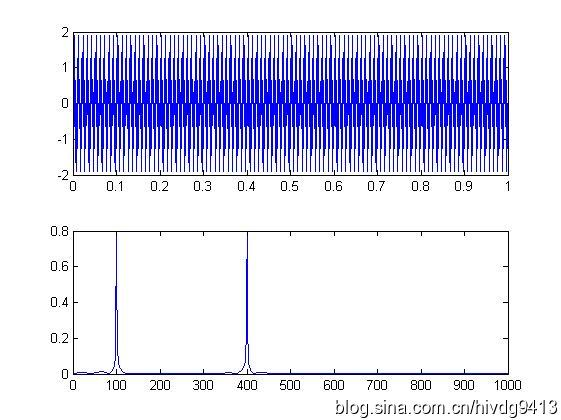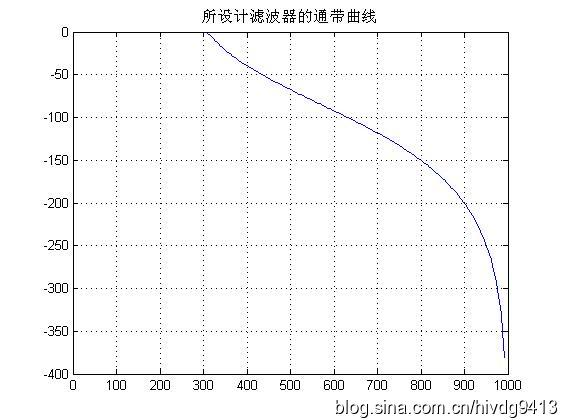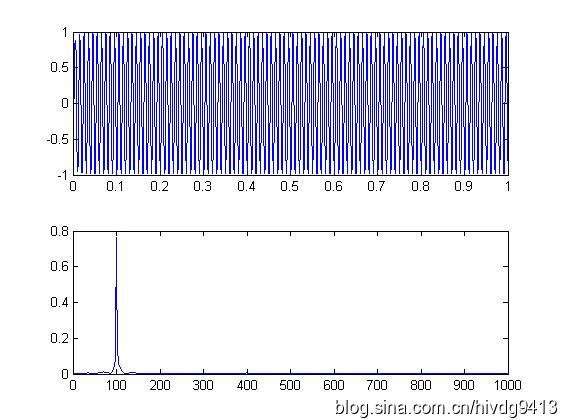2.高通滤波器function y=highp(x,f1,f3,rp,rs,Fs)
%高通滤波
%使用注意事项：通带或阻带的截止频率的选取范围是不能超过采样率的一半
%即，f1,f3的值都要小于 Fs/2
%x:需要带通滤波的序列
% f 1：通带截止频率
% f 2：阻带截止频率
%rp：边带区衰减DB数设置
%rs：截止区衰减DB数设置
%FS：序列x的采样频率
% rp=0.1;rs=30;%通带边衰减DB值和阻带边衰减DB值
% Fs=2000;%采样率
%
wp=2*pi*f1/Fs;
ws=2*pi*f3/Fs;
% 设计切比雪夫滤波器；
[n,wn]=cheb1ord(wp/pi,ws/pi,rp,rs);
[bz1,az1]=cheby1(n,rp,wp/pi,'high');

%查看设计滤波器的曲线
[h,w]=freqz(bz1,az1,256,Fs);
h=20*log10(abs(h));
figure;plot(w,h);title('所设计滤波器的通带曲线');grid on;
y=filter(bz1,az1,x);
end下面是高通滤波器的例子fs=2000;
t=(1:fs)/fs;
ff1=100;
ff2=400;
x=sin(2*pi*ff1*t)+sin(2*pi*ff2*t);
figure;
subplot(211);plot(t,x);
subplot(212);hua_fft(x,fs,1);

%------高通测试
z=highp(x,350,300,0.1,20,fs);
figure;
subplot(211);plot(t,z);
subplot(212);hua_fft(z,fs,1);下面三幅图分别是滤波前的时频图，滤波器的滤波特性曲线图和滤波后的时频图，通过图可以看出成功留下了400Hz的高频成分而把不要的低频成分100Hz去除了。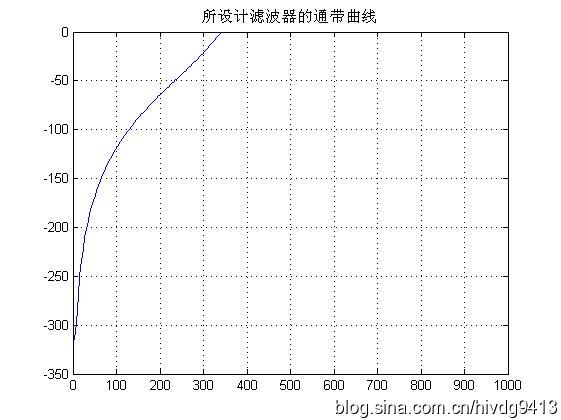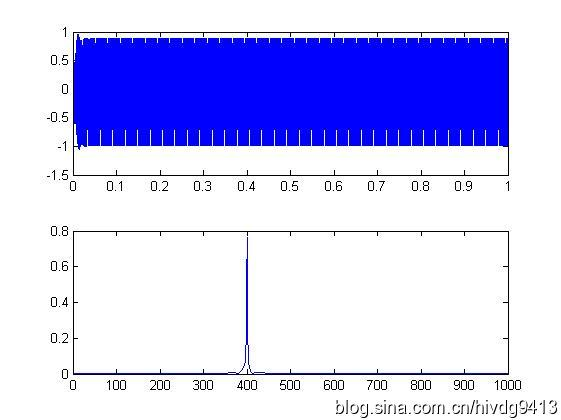3.带通滤波器function y=bandp(x,f1,f3,fsl,fsh,rp,rs,Fs)
%带通滤波
%使用注意事项：通带或阻带的截止频率与采样率的选取范围是不能超过采样率的一半
%即，f1,f3,fs1,fsh,的值小于 Fs/2
%x:需要带通滤波的序列
% f 1：通带左边界
% f 3：通带右边界
% fs1：衰减截止左边界
% fsh：衰变截止右边界
%rp：边带区衰减DB数设置
%rs：截止区衰减DB数设置
%FS：序列x的采样频率
% f1=300;f3=500;%通带截止频率上下限
% fsl=200;fsh=600;%阻带截止频率上下限
% rp=0.1;rs=30;%通带边衰减DB值和阻带边衰减DB值
% Fs=2000;%采样率
%
wp1=2*pi*f1/Fs;
wp3=2*pi*f3/Fs;
wsl=2*pi*fsl/Fs;
wsh=2*pi*fsh/Fs;
wp=[wp1 wp3];
ws=[wsl wsh];
%
% 设计切比雪夫滤波器；
[n,wn]=cheb1ord(ws/pi,wp/pi,rp,rs);
[bz1,az1]=cheby1(n,rp,wp/pi);
%查看设计滤波器的曲线
[h,w]=freqz(bz1,az1,256,Fs);
h=20*log10(abs(h));
figure;plot(w,h);title('所设计滤波器的通带曲线');grid on;
y=filter(bz1,az1,x);
end带通滤波器使用例子%--------------
%带通滤波器测试程序
fs=2000;
t=(1:fs)/fs;
ff1=100;
ff2=400;
ff3=700;
x=sin(2*pi*ff1*t)+sin(2*pi*ff2*t)+sin(2*pi*ff3*t);
figure;
subplot(211);plot(t,x);
subplot(212);hua_fft(x,fs,1);
% y=filter(bz1,az1,x);
y=bandp(x,300,500,200,600,0.1,30,fs);
figure;
subplot(211);plot(t,y);
subplot(212);hua_fft(y,fs,1);%调用到的hua_fft()函数代码如下function hua_fft(y,fs,style,varargin)
%当style=1,画幅值谱；当style=2,画功率谱;当style=其他的，那么花幅值谱和功率谱
%当style=1时，还可以多输入2个可选参数
%可选输入参数是用来控制需要查看的频率段的
%第一个是需要查看的频率段起点
%第二个是需要查看的频率段的终点
%其他style不具备可选输入参数，如果输入发生位置错误
nfft=2^nextpow2(length(y));%找出大于y的个数的最大的2的指数值（自动进算最佳FFT步长nfft）
%nfft=1024;%人为设置FFT的步长nfft
y=y-mean(y);%去除直流分量
y_ft=fft(y,nfft);%对y信号进行DFT，得到频率的幅值分布
y_p=y_ft.*conj(y_ft)/nfft;%conj()函数是求y函数的共轭复数，实数的共轭复数是他本身。
y_f=fs*(0:nfft/2-1)/nfft;�T变换后对应的频率的序列
% y_p=y_ft.*conj(y_ft)/nfft;%conj()函数是求y函数的共轭复数，实数的共轭复数是他本身。
if style==1
ifnargin==3
plot(y_f,2*abs(y_ft(1:nfft/2))/length(y));%matlab的帮助里画FFT的方法
%ylabel('幅值');xlabel('频率');title('信号幅值谱');
%plot(y_f,abs(y_ft(1:nfft/2)));%论坛上画FFT的方法
else
f1=varargin{1};
fn=varargin{2};
ni=round(f1 * nfft/fs+1);
na=round(fn * nfft/fs+1);
plot(y_f(ni:na),abs(y_ft(ni:na)*2/nfft));
end

elseif style==2
plot(y_f,y_p(1:nfft/2));
%ylabel('功率谱密度');xlabel('频率');title('信号功率谱');
else
subplot(211);plot(y_f,2*abs(y_ft(1:nfft/2))/length(y));
ylabel('幅值');xlabel('频率');title('信号幅值谱');
subplot(212);plot(y_f,y_p(1:nfft/2));
ylabel('功率谱密度');xlabel('频率');title('信号功率谱');
end
end运行结果如下图，第一幅是滤波前测试信号的时频图，第二幅是滤波器的滤波曲线图，第三幅是经滤波后的测试信号时频图。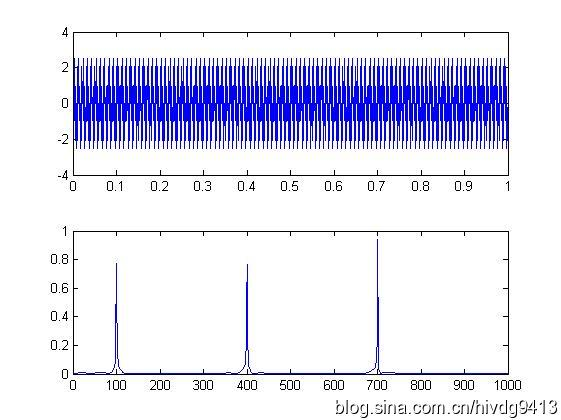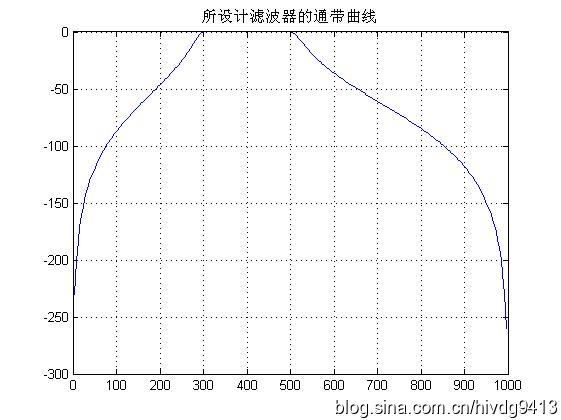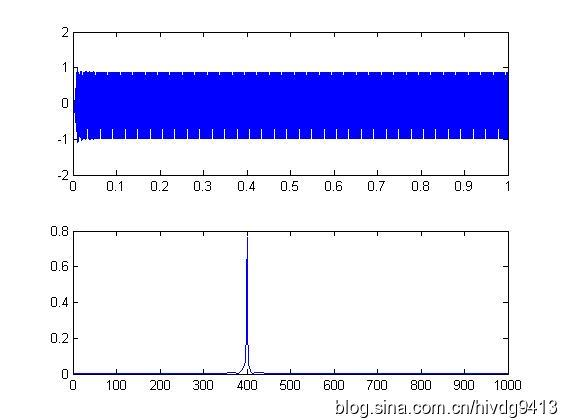4.带阻滤波器function y=bands(x,f1,f3,fsl,fsh,rp,rs,Fs)
%带阻滤波
%使用注意事项：通带或阻带的截止频率与采样率的选取范围是不能超过采样率的一半
%即，f1,f3,fs1,fsh,的值小于 Fs/2
%x:需要带通滤波的序列
% f 1：通带左边界
% f 3：通带右边界
% fs1：衰减截止左边界
% fsh：衰变截止右边界
%rp：边带区衰减DB数设置
%rs：截止区衰减DB数设置
%FS：序列x的采样频率
% f1=300;f3=500;%通带截止频率上下限
% fsl=200;fsh=600;%阻带截止频率上下限
% rp=0.1;rs=30;%通带边衰减DB值和阻带边衰减DB值
% Fs=2000;%采样率
%
wp1=2*pi*f1/Fs;
wp3=2*pi*f3/Fs;
wsl=2*pi*fsl/Fs;
wsh=2*pi*fsh/Fs;
wp=[wp1 wp3];
ws=[wsl wsh];
%
% 设计切比雪夫滤波器；
[n,wn]=cheb1ord(ws/pi,wp/pi,rp,rs);
[bz1,az1]=cheby1(n,rp,wp/pi,'stop');
%查看设计滤波器的曲线
[h,w]=freqz(bz1,az1,256,Fs);
h=20*log10(abs(h));
figure;plot(w,h);title('所设计滤波器的通带曲线');grid on;
y=filter(bz1,az1,x);
end使用例子%带阻滤波器测试
fs=1000;
t=(1:fs)/fs;
y=sin(2*pi*100*t)+sin(2*pi*150*t)+sin(2*pi*200*t);
figure;hua_fft(y,fs,1);
z=bands(y,110,190,140,160,0.1,30,fs);
figure;hua_fft(z,fs,1);运行结果如下图，第一幅是滤波前测试信号的频谱图，第二幅是滤波器的滤波曲线图，第三幅是经滤波后的测试信号频谱图。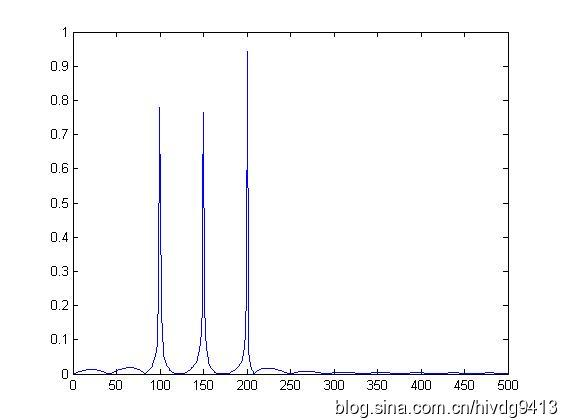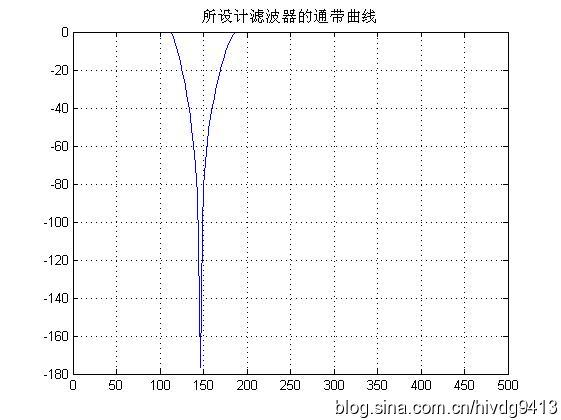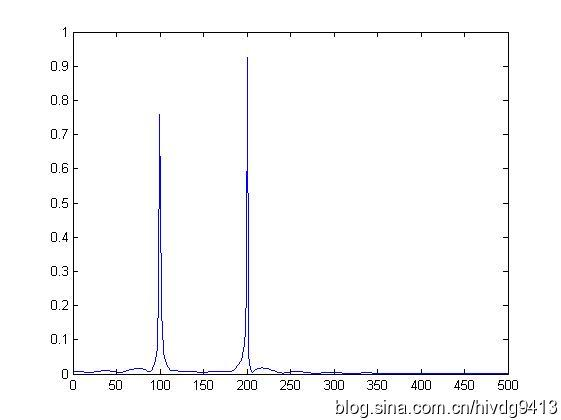展开全文低通滤波器 高通滤波器 带通滤波器 带阻滤波器 matlab
• ## matlab设计模拟带通滤波器

万次阅读 多人点赞 2018-10-25 12:44:02
简单记录下在matlab上如何设计出模拟的带通滤波器，包括：巴特沃斯滤波器、切比雪夫I型滤波器、切比雪夫II型滤波器、椭圆型滤波器。 代码如下： %设计带通滤波器 %巴特沃斯、切比雪夫I型、切比雪夫II型、椭圆型...
简单记录下在matlab上如何设计出模拟的带通滤波器，包括：巴特沃斯滤波器、切比雪夫I型滤波器、切比雪夫II型滤波器、椭圆型滤波器。
代码如下：
%设计带通滤波器
%巴特沃斯、切比雪夫I型、切比雪夫II型、椭圆型滤波器

clear all;

%wp和ws分别是通带和阻带的频率(截止频率)。当wp和ws为二元矢量时，为带通或带阻滤波器，这时求出的Wn也是二元矢量；当wp和ws为一元矢量时，为低通或高通滤波器：当wp<ws时为低通滤波器，当wp>ws时为高通滤波器。

%wp和ws为二元矢量
wp=[0.1*2*pi 0.15*2*pi];                %设置通带频率
ws=[0.05*2*pi 0.2*2*pi];                %设置阻带频率

Rp=1;                                   %设置通带波纹系数
Rs=20;                                  %设置阻带波纹系数

%巴特沃斯滤波器设计
[N,Wn]=buttord(wp,ws,Rp,Rs,'s');        %求巴特沃斯滤波器阶数，输出参数N代表满足设计要求的滤波器的最小阶数，Wn是等效低通滤波器的截止频率
%无论是高通、带通和带阻滤波器，在设计中最终都等效于一个截止频率为Wn的低通滤波器(我现在也不是很理解为啥是这样，毕竟我也是刚接触滤波器)
fprintf('巴特沃斯滤波器 N= %4d\n',N);    %显示滤波器阶数
[bb,ab]=butter(N,Wn,'s');               %求巴特沃斯滤波器系数，即求传输函数的分子和分母的系数向量
W=0:0.01:2;                             %设置模拟频率
[Hb,wb]=freqs(bb,ab,W);                 %求巴特沃斯滤波器频率响应
plot(wb/pi,20*log10(abs(Hb)),'b');      %作图
hold on

%切比雪夫I型滤波器设计
[N,Wn]=cheb1ord(wp,ws,Rp,Rs,'s');       %求切比雪夫I型滤波器阶数
fprintf('切比雪夫I型滤波器 N= %4d\n',N); %显示滤波器阶数
[bc1,ac1]=cheby1(N,Rp,Wn,'s');          %求切比雪夫I型滤波器系数，即求传输函数的分子和分母的系数向量
[Hc1,wc1]=freqs(bc1,ac1,W);             %求切比雪夫I型滤波器频率响应
plot(wc1/pi,20*log10(abs(Hc1)),'k');    %作图

%切比雪夫II型滤波器设计
[N,Wn]=cheb2ord(wp,ws,Rp,Rs,'s');       %求切比雪夫II型滤波器阶数
fprintf('切比雪夫II型滤波器 N= %4d\n',N);%显示滤波器阶数
[bc2,ac2]=cheby2(N,Rs,Wn,'s');          %求切比雪夫II型滤波器系数，即求传输函数的分子和分母的系数向量
[Hc2,wc2]=freqs(bc2,ac2,W);             %求切比雪夫II型滤波器频率响应
plot(wc2/pi,20*log10(abs(Hc2)),'r');    %作图

%椭圆型滤波器设计
[N,Wn]=ellipord(wp,ws,Rp,Rs,'s');       %求椭圆型滤波器阶数
fprintf('椭圆型滤波器 N= %4d\n',N);      %显示滤波器阶数
[be,ae]=ellip(N,Rp,Rs,Wn,'s');          %求椭圆型滤波器系数，即求传输函数的分子和分母的系数向量
[He,we]=freqs(be,ae,W);                 %求椭圆型滤波器频率响应
%作图
plot(we/pi,20*log10(abs(He)),'g');
axis([0 max(we/pi) -30 2]);
legend('巴特沃斯滤波器','切比雪夫I型滤波器','切比雪夫II型滤波器','椭圆型滤波器');
xlabel('角频率{\omega}/{\pi}');
ylabel('幅值/dB');
line([0 max(we/pi)],[-20 -20],'color','k','linestyle','--');%在画布上画线
line([0 max(we/pi)],[-1 -1],'color','k','linestyle','--');
line([0.2 0.2],[-30 2],'color','k','linestyle','--');
line([0.3 0.3],[-30 2],'color','k','linestyle','--');

运行结果如下：
巴特沃斯滤波器 N=    4
切比雪夫I型滤波器 N=    3
切比雪夫II型滤波器 N=    3
椭圆型滤波器 N=    2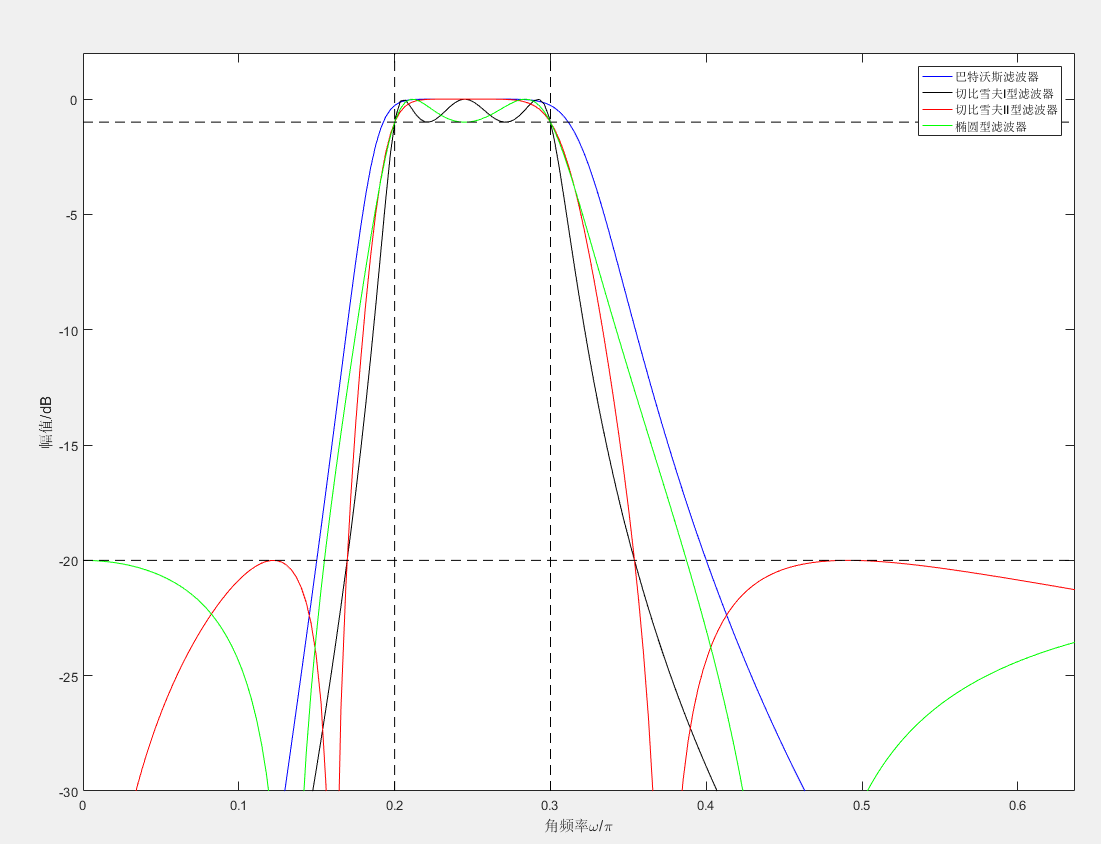注：
1、求各种滤波器的传输函数的分子和分母向量系数的函数，例如：
[bc1,ac1]=cheby1(N,Rp,Wn,'s');          %求切比雪夫I型滤波器系数，即求传输函数的分子和分母的系数向量

这里的函数cheby1()中的参数为
cheby1(N,Rp,Wn,'type','s');

其中注意’type’可为高通：'high’或带阻：‘stop’，如果不填的话，默认是低通或者带通，至于是低通还是带通，主要看你前面的wp和ws是二元矢量还是一元矢量：二元矢量对应带通，一元矢量对应低通。
2、matlab中的line()函数简单介绍：
https://blog.csdn.net/qq_36554582/article/details/83352155


展开全文• 学习MATLAB的filter函数的使用，通过设计低通、带通和高通滤波器对其进行仿真 2、用到的主要函数和工具 MATLAB、FDATOOL、filter、fft 3、设计 信号的产生 %% Parameter Interface Frequence0 = 60; %单位：Hz ...
1、目的

学习MATLAB的filter函数的使用，通过设计低通、带通和高通滤波器对其进行仿真

2、用到的主要函数和工具

MATLAB、FDATOOL、filter、fft

3、设计

信号的产生
%% Parameter Interface
Frequence0          = 60;        %单位：Hz
Frequence1          = 130;       %单位：Hz
Frequence2          = 1e3;       %单位：Hz
SampleFre           = 4e3;       %单位：Hz
SampleLen           = SampleFre; %采样点数
%% Main
%-------------------产生三路信号
t = 0:1/SampleLen:1/SampleFre*(SampleLen-1);
SignalData0 = sin(2*pi*Frequence0*t);
SignalData1 = sin(2*pi*Frequence1*t);
SignalData2 = sin(2*pi*Frequence2*t);
SignalData3 = SignalData0+SignalData1+SignalData2;
figure;hold on
plot(t(1:150),SignalData0(1:150),'b')
plot(t(1:150),SignalData1(1:150),'r')
plot(t(1:150),SignalData2(1:150),'k')
hold off
figure;plot(t(1:150),SignalData3(1:150))
title('三路信号求和')

三路信号的时域波形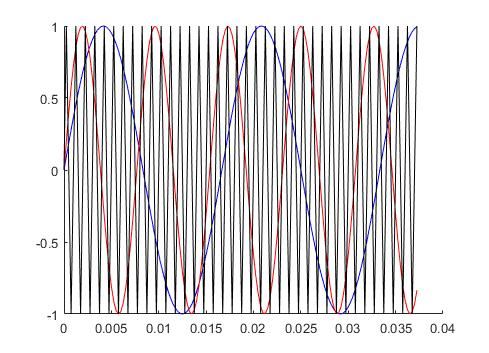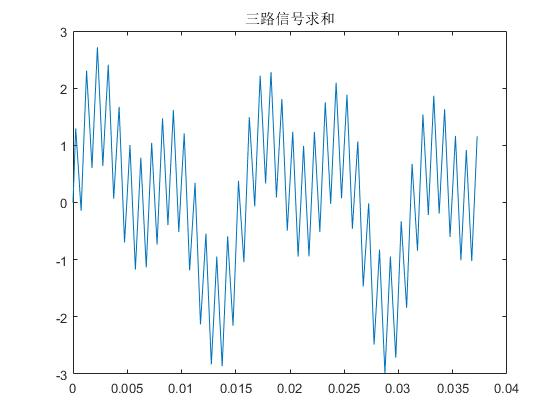三路信号时域波形                                                                      三路信号求和时域波形

三路求和信号频谱分析
FFT_Data = fft(SignalData3);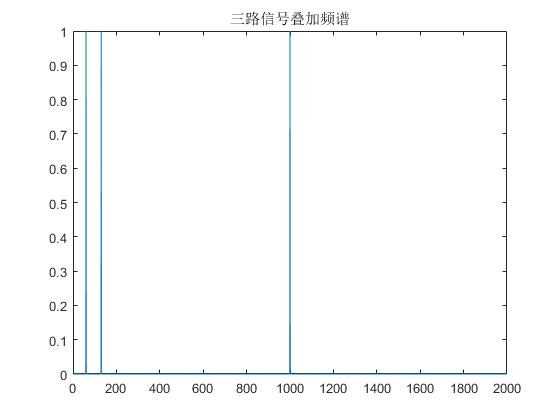2.低通滤波器设计

基于fdatool的低通滤波器的设计
在MATLAB命令行执行fdatool命令，弹出滤波器设计交互窗口，根据自己的需求设计符合自己要求的滤波器，这是我设计的低通滤波器见下图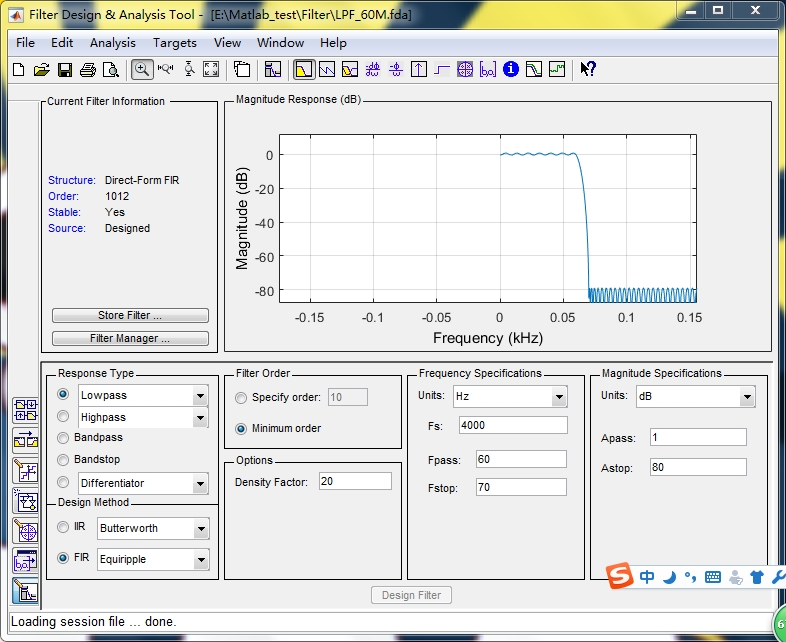需要注意的是通过设计Fpass和Fstop确定了滤波器的带宽，同时参数设计的越接近理论滤波器的性能，滤波器的阶数越多，计算量越大，所以需要优化滤波器这两个参数，在保证性能的同时确保阶数越小（计算量越小）

滤波
LPF_Data = filter(LPF_Coe.LPF_60M,1,SignalData3);

滤波后的时域和频谱波形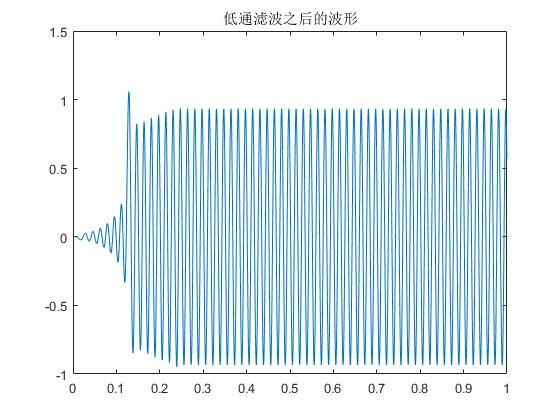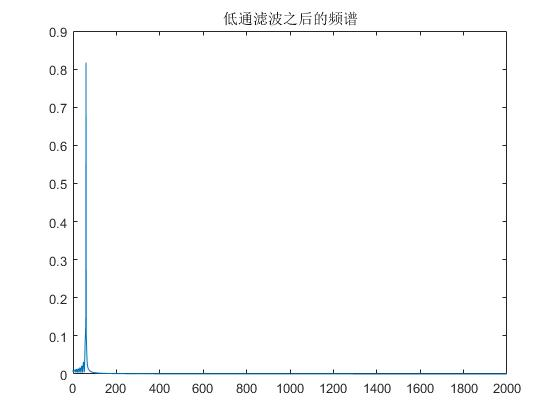3.带通和高通操作步骤大体一致就不详细说明

4、M代码

5、备注

1、滤波器出数有一定延迟，这在具体调制解调过程中需要注意
展开全文• FilterPro是TI公司出品的一个有源滤波器设计的工具，十分好用，作为电子相关滤波器设计是十分必要的。 大致过下流程，首先选择设计什么滤波器，低通，高通，带通，带阻，
http://download.csdn.net/detail/fzxy002763/3649600
FilterPro是TI公司出品的一个有源滤波器设计的工具，十分好用，作为电子相关滤波器设计是十分必要的。
大致过下流程，首先选择设计什么滤波器，低通，高通，带通，带阻，还有全通。第二部设置一些具体的参数，增益，通带频率，纹波系数，阻带频率，带外衰减，还有就是滤波器阶数第三部滤波器类型选择，这里有很多种类似对比的参数图，可以根据不同需要选择如贝塞尔，巴特沃斯等滤波器，其实一般选巴特沃斯就够了，比较好实现一些。第四部，选择滤波器的拓扑结构，有反馈(Multiple-Feedback)实际还分为单路和差分两种,还有Sallen-key(Q值越大截止频率越抖)这几种类型的。基本设置做完了，滤波器就自动生成了，如图：这里还可以看到一些具体设计出来的滤波器参数，可以对照一下看看是不是满足设计的需求。其他的还有一些相关的具体滤波器的参数信息，做一些具体的报告的时候用得上，还有一些滤波器电阻电容电感这些器件的清单。这里还有一些注意的就是关于放大器的选择，一般实际上NE5532之类的都可以了，比如说OPA637之类的比较高级的放大器有时候效果还没有低端一些的好，自己测试得出的一些经验。

展开全文工具 测试
• 滤波器主要使用频率范围参考通过相应数据手册或规格书！！！   低通滤波器：SLFL31-0R530G-01TF 通过参数...带通滤波器：HDF475C，它是一个表征滤波器，通过手册可以发现它的本质是带通滤波器。 中心频率为4...
• ## MATLAB实现巴特沃斯数字滤波器

万次阅读 多人点赞 2019-08-10 13:55:09
MATLAB实现巴特沃斯数字滤波器 MATLAB实现巴特沃斯数字滤波器 前因：因为要准备保研面试，今年暑假就重新把烂尾的项目捡起来了。 为了提取采集到的脑电信号中有用的部分，想用数字带通滤波器实现，浏览了很多帖子...MATLAB 带通滤波
• MATLAB IIR数字滤波器设计   首先我们要明白相关的概念。 数字滤波器设计采用角频率，如何与实际信号频率对应？ 角频率w，采样频率fs ,实际信号...当我们设计的滤波器带通的时候。其通带截止频率有两个，阻带截
• FDATOOL界面如图所示，总共分两大部分：一部分是Design Filter，在界面的下半部，用来设置滤波器的设计参数；另一部分则是特性区，在界面的上半部分，用来显示滤波器的各种特性。 Design Filter部分主要分为...
• 参数b决定是普通滤波器还是带通滤波器 小波变换前先进行卡尔曼平滑滤波 小波变换的作用： [c,l] = wavedec(y(:,i),3,'db4'); wavedec函数用于一维小波变换，对信号进行多层分解 [c,l]=wavedec(x,N,’wname...
• 看很多人推荐用书 《基于MATLAB的系统分析与设计...通俗点说截止频率就是指一个系统的输出信号能量开始大幅下降或者在带阻滤波器中为大幅上升的边界频率（一般以-3dB为界限），-3dB衰减是指20*log（输出/输入）=-3d...数字滤波器的设计
• FIR 滤波器广泛应用于数字信号处理中，主要功能就是将不感兴趣的信号滤除，留下有用信号。FIR滤波器是全零点结构，系统永远稳定；并且具有线性相位的特征，在有效频率范围内所有信号相位上不失真。在无线通信收发机...
• 一直对信号分析与处理有着比较浓厚的兴趣，只可惜...实现的功能是将三个幅度都为1初相位0，频率分别为10Hz、20Hz、30Hz的正弦信号叠加在一起，将混叠后的信号通过一个带通滤波器，只剩下20Hz的正弦信号，用示波器显示
• IIR滤波器的MATLAB设计 1 FDATool界面设计 1.1 FDATool的介绍  FDATool(Filter Design&Analysis Tool)是MATLAB信号处理工具箱里专用的滤波器设计分析工具，MATLAB 6.O以上的版本还专门增加了滤波器设计工具箱...
• ## Matlab滤波器设计

万次阅读 2015-01-07 15:09:43
滤波器设计是一个创建满足指定滤波要求的滤波器参数的过程。滤波器的实现包括滤波器结构的选择和滤波器参数的计算。只有完成了滤波器的设计和实现，才能最终完成数据的滤波。  滤波器设计的目标是实现数据序列的...matlab filter 工具 vector hp
• bandpass带通滤波详解基本...先上代码，下面是带通滤波器在matlab中的使用 function [ pc ] = m_bandpass( pc ) freq = [0.1 0.5]; fs= 50; % Fa=Fs/2，Fa是分析频率 [b,a] = butter(3,freq/(fs/2),'ban...
• ## Python实现FIR带通滤波器

千次阅读 热门讨论 2020-05-25 23:31:54
1、FIR算法实现 y(0)=∑0Nh(i)x(i)y(0)=\sum _{0}^Nh(i)x(i)y(0)=∑0N​h(i)x(i) class filter: def __init__(self,order,h): self.order=order self.h=h self.output=[] def FIR_Filter(se...Python
• ## FDAT设计滤波器方法

千次阅读 2012-07-25 13:06:11
IIR滤波器的MATLAB设计 1 FDATool界面设计 1.1 FDATool的介绍  FDATool(Filter Design&Analysis Tool)是MATLAB信号处理工具箱里专用的滤波器设计分析工具，MATLAB 6.O以上的版本还专门增加了滤波器设计工具箱...matlab filter processing 工具 signal
• 滤波器选择和使用注意事项 1. 如何选择电源线干扰滤波器？ 有的顾客以为EMI滤波器的插入损耗越高越好，滤波网络的级数越多越好。其实这并不是选择滤波器的正确方法。此外，级数越多的滤波网络，价格越贵，体积和...
• 然后按照下图设置相关参数参数列表中的Fs应该是采样频率，Fc是截止频率，即幅频特性曲线-3dB对应的频率。点击“Design Filter”后，幅频特性曲线会被更新。然后切换到下面这个页面，点击“Realize Model”后，会...
...